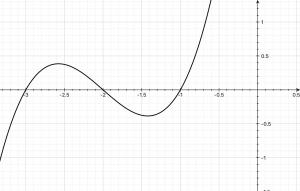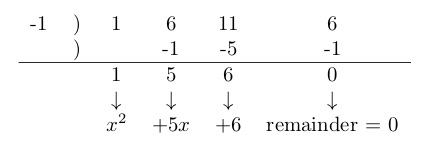# Rational Roots Test

## What is the rational roots test?

The rational roots test is a tool for finding zeros in polynomial functions that have rational zeros (roots). Not every polynomial function has rational zeros*, but you can’t usually tell just by looking. The rational roots test can help you narrow down a function’s possible rational roots.

*Note: A zero is the x-value where a graph crosses the y-axis — that is, where the function’s value is zero. It can also be called a root, an x-intercept, or a solution.

## Why does it work?

To apply a theorem you usually don’t need to know why it works. But it may help.

The rational roots theorem says that if a polynomial

has rational roots, those roots will be limited to  where p is a factor of  and q is a factor of .

For example, suppose you are given a polynomial function  and asked to find its rational zeros. To apply the rational roots test, you need consider only the first term,  , and the last, 6. The last term, -6, is p. Its only factors are  -1, 1, -2, 2, -3, 3, -6 and 6. The coefficient of the leading term, 1, is q. Its only factors are and Then the only possible zeros are factors of p divided by factors of q: -1, 1, -2, 2, -3, 3, -6 and 6.

.

The factor theorem says that if a polynomial has a factor then c is a zero of that polynomial. The polynomial equals zero when any of its factors equal zero, so

Then  is a zero.

Let’s ramp it up. Say and  and maybe a bunch more like that are factors. You may get this problem in the form   and be asked to find the zeros. Note that is what you get when you multiply all the q‘s and is what you get when you multiply all the p‘s. Your clue is that each rational zero is some factor of divided by some factor of . You may not be able to sort out all those p‘s and q‘s, but you can narrow down the possibilities: For rational zeros, p’s are limited to the factors of the constant term and q’s are limited to the factors of the leading coefficient.

## Example

Consider the function

# .

What are its roots?

Per the rational roots test, the possible zeros are the positive and negative of the factors of the constant term, 6 in this case, divided by factors of the leading coefficient, 1 in this case.

As shown above, those numbers are -1, 1, -2, 2, -3, 3, -6, and 6. How you can narrow down the possibilities depends to some extent on the rules in the class you are taking. My own preferred method is to graph the function and see what seem to be the roots.

Here is a graph ofKeep in mind that what looks like a zero in a graph may not be a zero. The graph just isn’t that precise – it may be off by just a little, too little to see. So when you see what looks like a zero, you need to confirm it.

This graph seems to show three zeros: -1, -2, and -3. One way to check out a possible zero is to substitute each apparent root into the original function. For example, try x=-1. Then Check. That means -1 is a root and is a factor.

Another way to check out a possible zero is synthetic division or polynomial long division, if you prefer. Let’s try -1 again this way. That looks like this:The remainder is zero; that confirms that -1 is a zero and is a root.

Once you get down to the second degree level, you can factor. That’s probably easier than continuing to use synthetic division. Use the depressed equation that results from synthetic division — or the quotient you get from polynomial long division. For this example that’s . That factors to and and  Those are the other two zeros that show up on the graph.

If you don’t have access to a graphing calculator, you can still try possible values you find of zeros. To minimize your effort, start with easy ones like 1 and -1.

As stated above, not every polynomial has rational roots. You may find no rational factors because, well there may not be any.